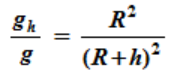# Acceleration Due to Gravity at different Altitudes Calculator

This CalcTown calculator calculates the acceleration due to gravity at a height h from the surface of the Earth.

Km

#### Result

ms-2where

gh = Acceleration at a height h

g=Acceleration on the surface of Earth = 9.8 ms-2

R=Radius of Earth=6,400 Km

h=Height above the surface at which the value of acceleration is to be measured.